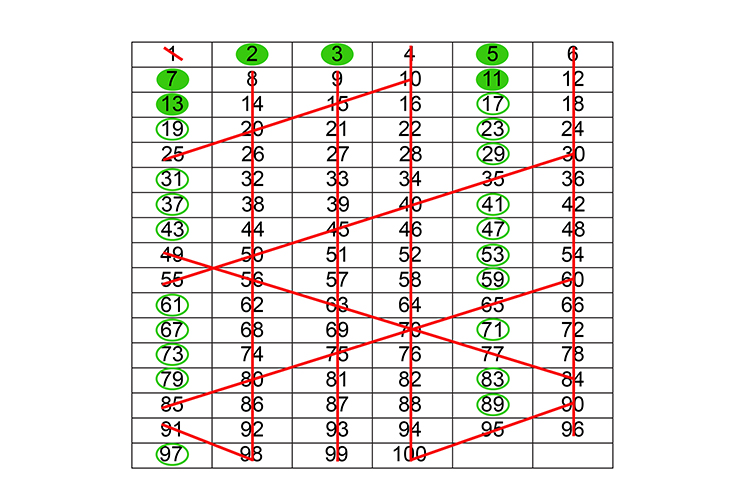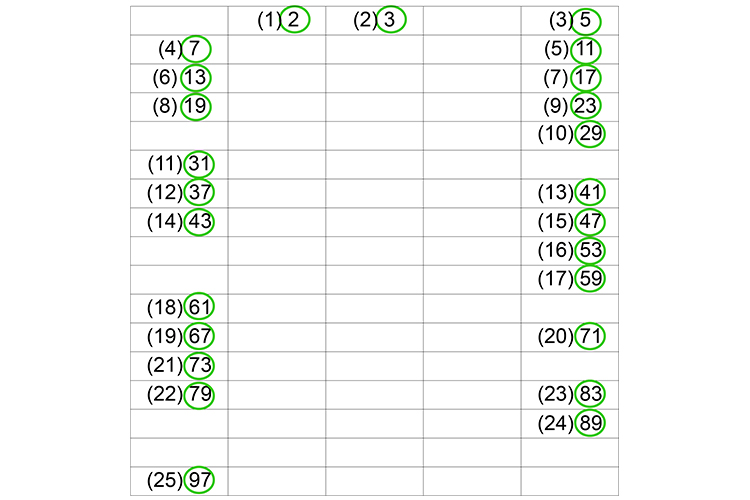# Finding all prime numbers between 1 to 100

To check remember:

1 to 100=25 Prime numbers

There are only 25 prime numbers between 1 and 100.## Rules

1.  Write all the numbers from 1 to 100 in six columns.

2.  Scratch out the 1 – it's not a prime number.

3.  Circle the ones you do know

 My dog bites several K G B men 2 3 5 7 1 1 1 3
 2 3 5 7 11 13

4.  Now draw a straight line down all the even numbers except 2.

5.  Draw a straight line down all the multiples of three except 3.

6.  Draw diagonal lines for all the multiples of 5 and 7 except 5 and 7.

This leaves the 25 prime numbers between 1 and 100 which are: